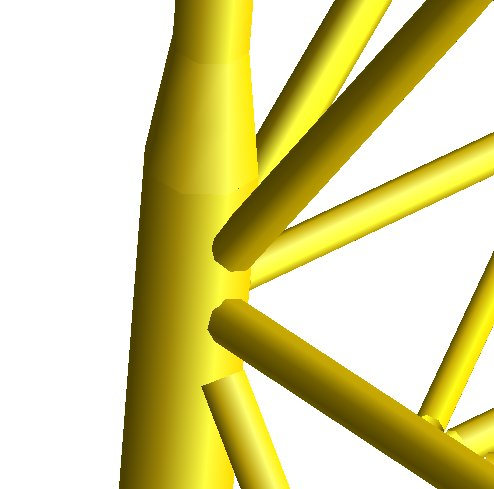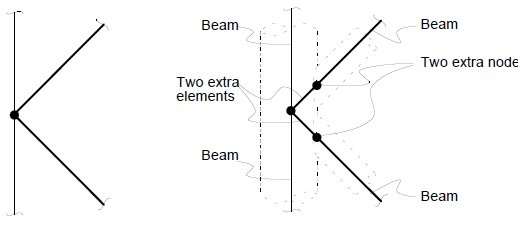Usfos Joint Examples.For many strucutres, the tubular joint connections (the welded connection between the brace and the chord) are weaker than the basic members. Assuming node-node connected elements is non-conservative for such structures. In order to describe the tubular joint behaviour correctly, the following modeling is needed:

• One element for the brace-member (conventional beam-column element)
• One element for the brace-chord connection (special element)

Figure 1 describes how Usfos automatically modifies the conventional Node-Node model to a model with two extra nodes and elements.Figure 1 : Users model (left) and the model modified by Usfos (two extra nodes and elements).

Usfos has different models for the tubular joint behaviour:

• Linear Elastic Joint Flexibility element. (the SHELL option)
• Non linear Joint Strength formulations (The CHJOINT option according to ISO, API, NORSOK formulaitons)